# programming 1 Introduction to Object Oriented Programming 1434

• Slides: 43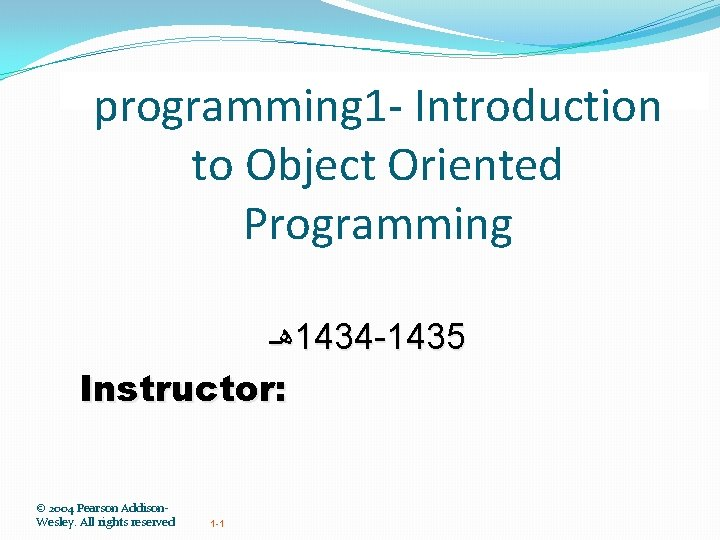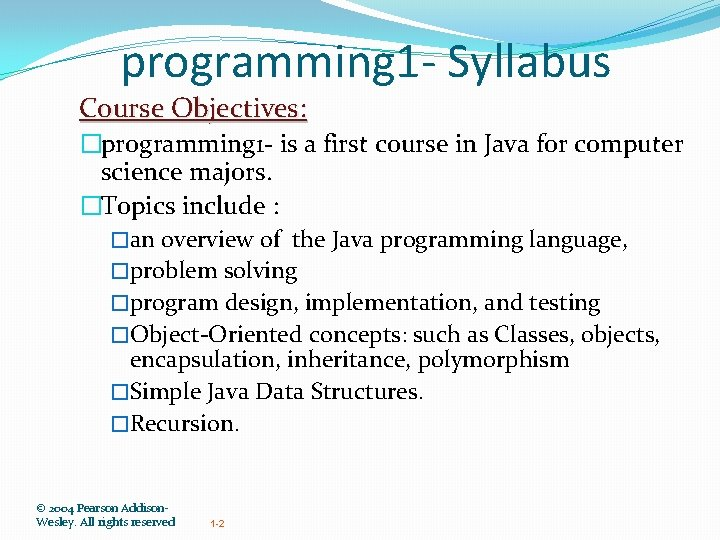programming 1 - Syllabus Course Objectives: �programming 1 - is a first course in Java for computer science majors. �Topics include : �an overview of the Java programming language, �problem solving �program design, implementation, and testing �Object-Oriented concepts: such as Classes, objects, encapsulation, inheritance, polymorphism �Simple Java Data Structures. �Recursion. © 2004 Pearson Addison. Wesley. All rights reserved 1 -2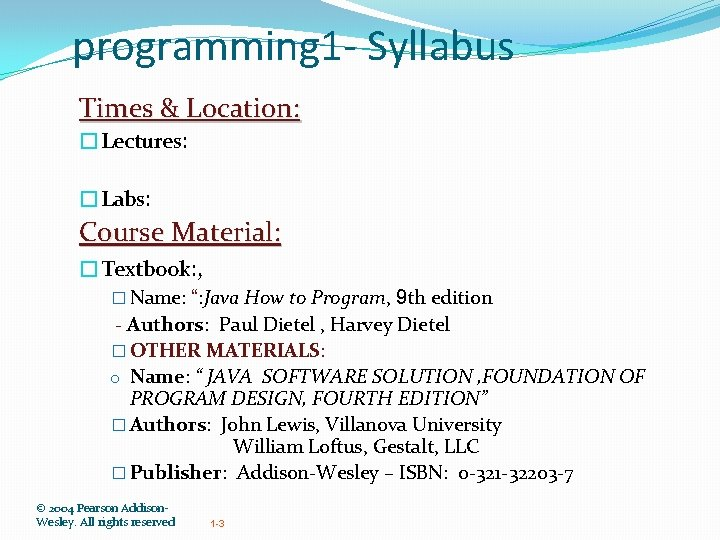programming 1 - Syllabus Times & Location: �Lectures: �Labs: Course Material: �Textbook: , � Name: “: Java How to Program, 9 th edition - Authors: Paul Dietel , Harvey Dietel � OTHER MATERIALS: o Name: “ JAVA SOFTWARE SOLUTION , FOUNDATION OF PROGRAM DESIGN, FOURTH EDITION” � Authors: John Lewis, Villanova University William Loftus, Gestalt, LLC � Publisher: Addison-Wesley – ISBN: 0 -321 -32203 -7 © 2004 Pearson Addison. Wesley. All rights reserved 1 -3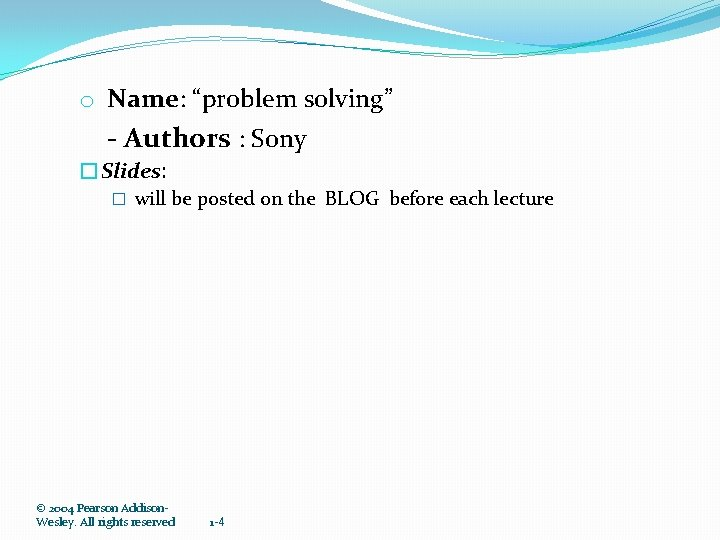o Name: “problem solving” - Authors : Sony �Slides: � will be posted on the BLOG before each lecture © 2004 Pearson Addison. Wesley. All rights reserved 1 -4programming 1 - Syllabus Office hours: Saturday Sunday Monday Tuesday Other : By appointment & by email Grading Policy : 10% 30% 10% 40% Exam 1 Exam 2 Lab: Exercises & Attendance & Quizzes Programming Assignments Final exam Communication Policy: � apply to join the class group at : � BLOG OF SUBJECT : CCIS 141. WORDPRESS. COM � &BLACKBOARD © 2004 Pearson Addison. Wesley. All rights reserved 1 -5programming 1 - Syllabus Coursework �Reading. � Students are responsible for reading and understanding the material in the textbook. However, we will not necessarily cover all of it in class. � If you do not understand something in the book, there will be opportunity to ask questions in class, during your teachers' office hours. �Programming: � There will be a lab programming exercises which will be given in the lab and due the next lab period. � Also there will be programming assignments which you will do at home. �Due Dates: � Labs are due the next lab period. � Programming assignments will be due the assigned date. © 2004 Pearson Addison. Wesley. All rights reserved 1 -6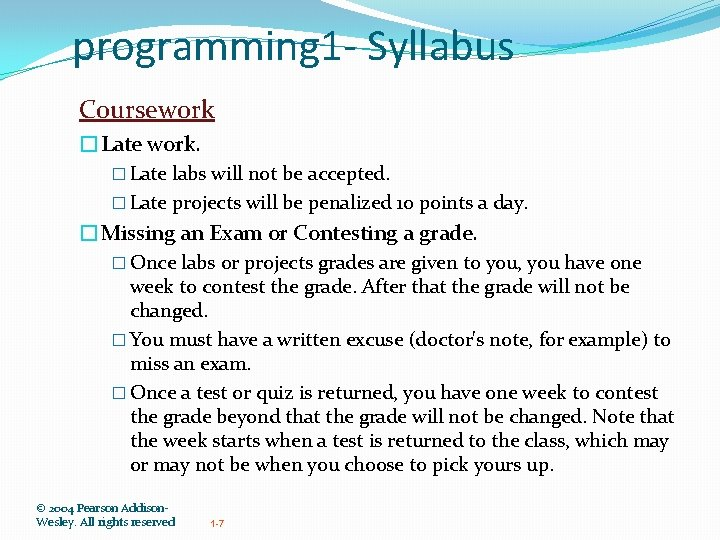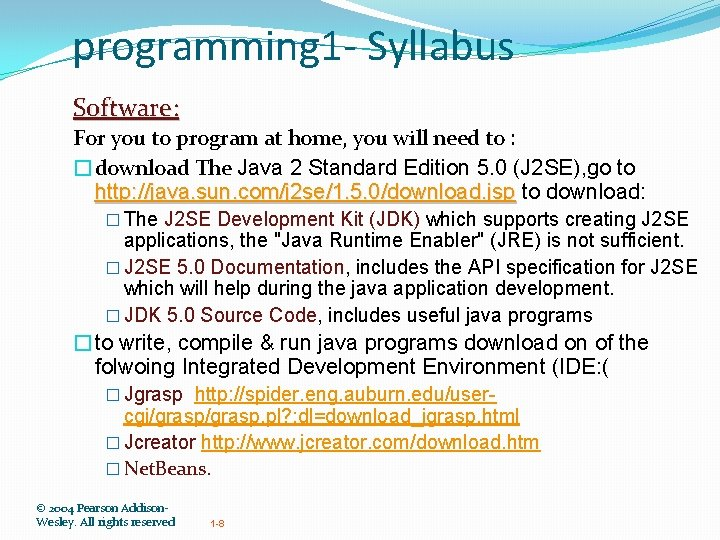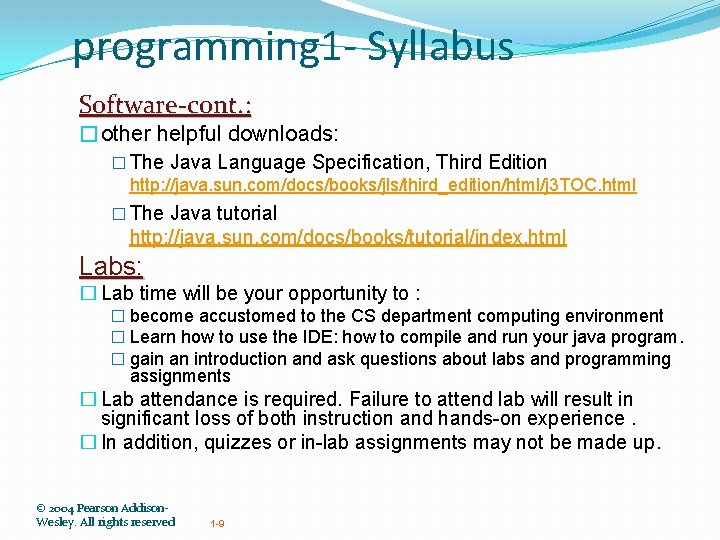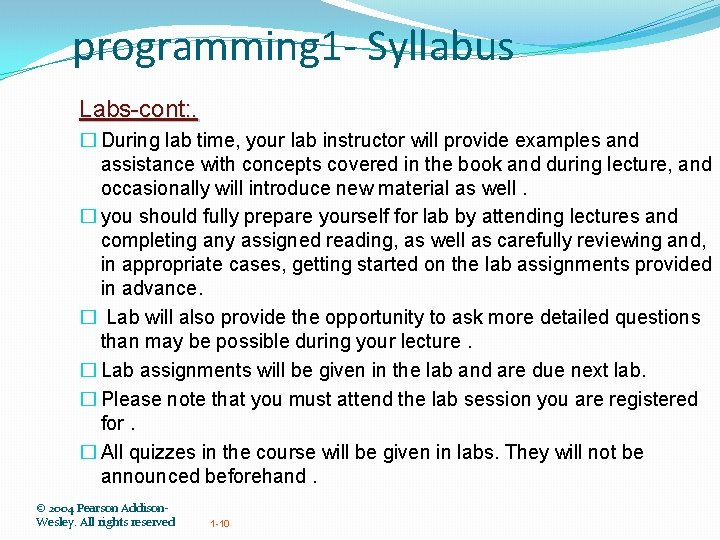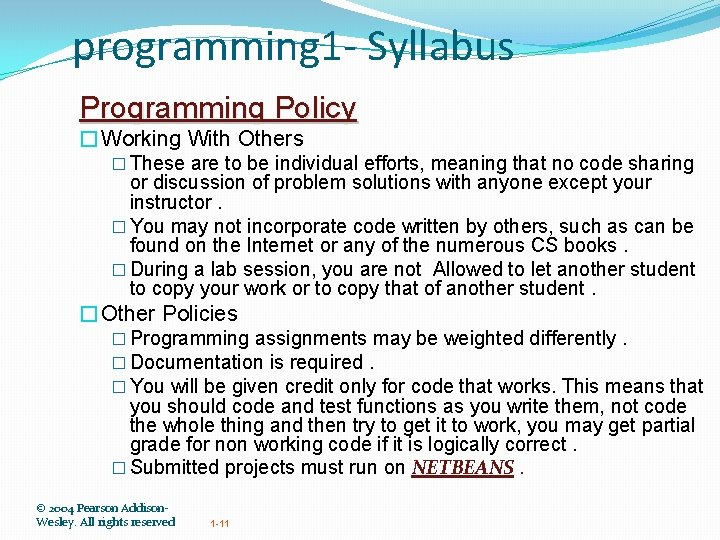programming 1 - Syllabus Programming Policy �Working With Others � These are to be individual efforts, meaning that no code sharing or discussion of problem solutions with anyone except your instructor. � You may not incorporate code written by others, such as can be found on the Internet or any of the numerous CS books. � During a lab session, you are not Allowed to let another student to copy your work or to copy that of another student. �Other Policies � Programming assignments may be weighted differently. � Documentation is required. � You will be given credit only for code that works. This means that you should code and test functions as you write them, not code the whole thing and then try to get it to work, you may get partial grade for non working code if it is logically correct. � Submitted projects must run on NETBEANS. © 2004 Pearson Addison. Wesley. All rights reserved 1 -11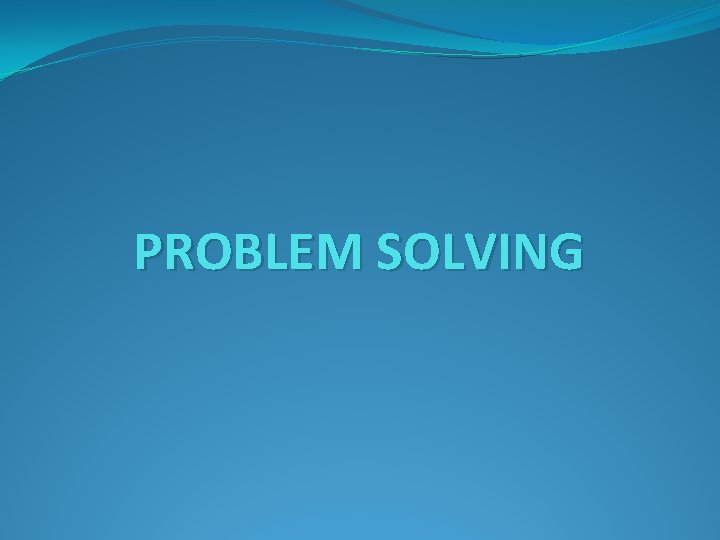PROBLEM SOLVING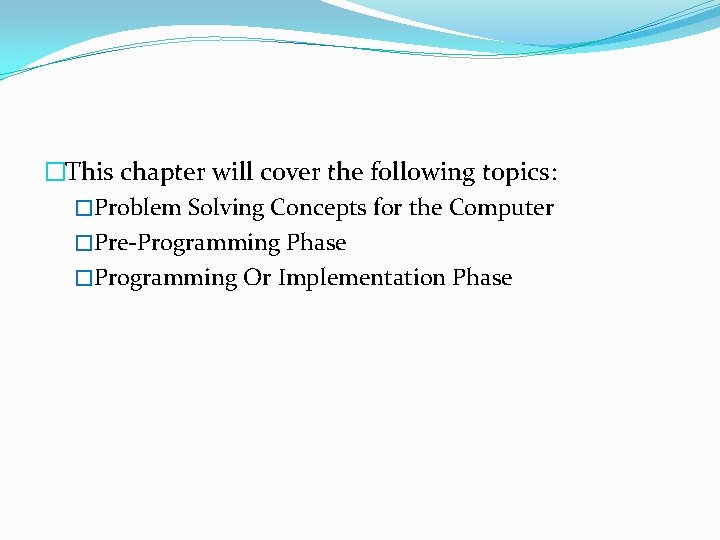�This chapter will cover the following topics: �Problem Solving Concepts for the Computer �Pre-Programming Phase �Programming Or Implementation Phase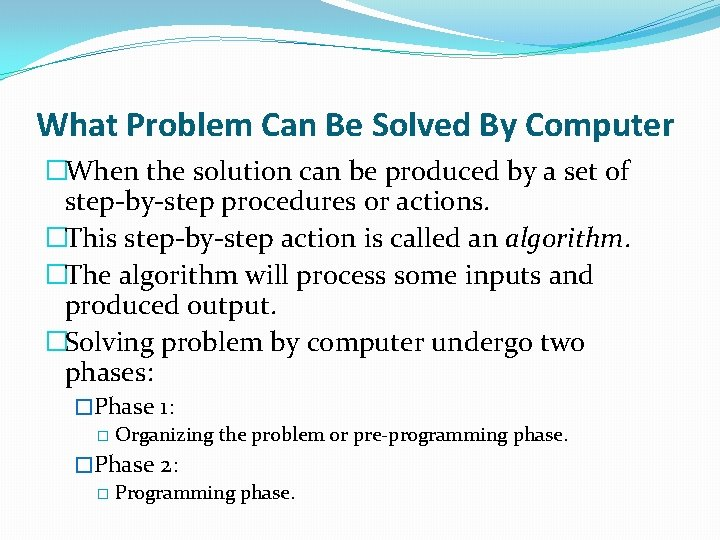What Problem Can Be Solved By Computer �When the solution can be produced by a set of step-by-step procedures or actions. �This step-by-step action is called an algorithm. �The algorithm will process some inputs and produced output. �Solving problem by computer undergo two phases: �Phase 1: � Organizing the problem or pre-programming phase. �Phase 2: � Programming phase.PRE-PROGRAMMING PHASE This phase requires five steps: 1. 2. 3. 4. 5. Analyzing the problem - PAC Developing the Hierarchy Input Process Output (HIPO) chart or Interactivity Chart (IC). Developing the Input-Process-Output (IPO) Chart. Drawing the Program flowcharts. Writing the algorithms.PRE-PROGRAMMING PHASE 1. Analyzing The Problem �Understand analyze the problem to determine whether it can be solved by a computer. �Analyze the requirements of the problem. �Identify the following: � Data requirement. � Processing requirement or procedures that will be needed to solve the problem. � The output.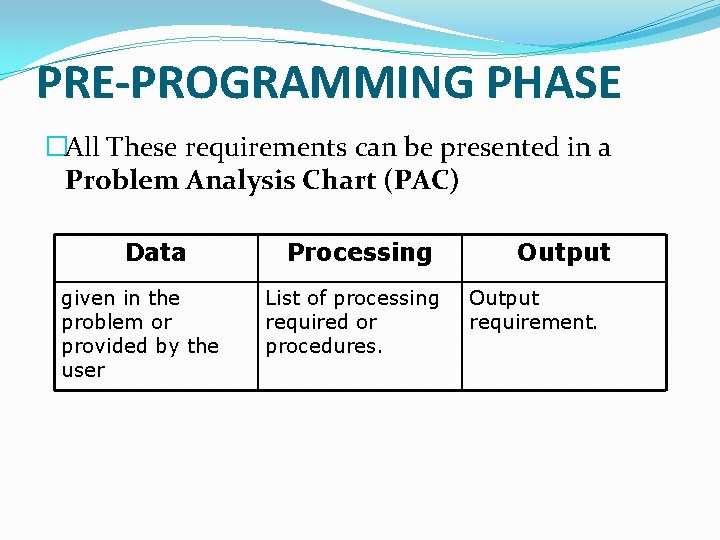PRE-PROGRAMMING PHASE �All These requirements can be presented in a Problem Analysis Chart (PAC) Data given in the problem or provided by the user Processing List of processing required or procedures. Output requirement.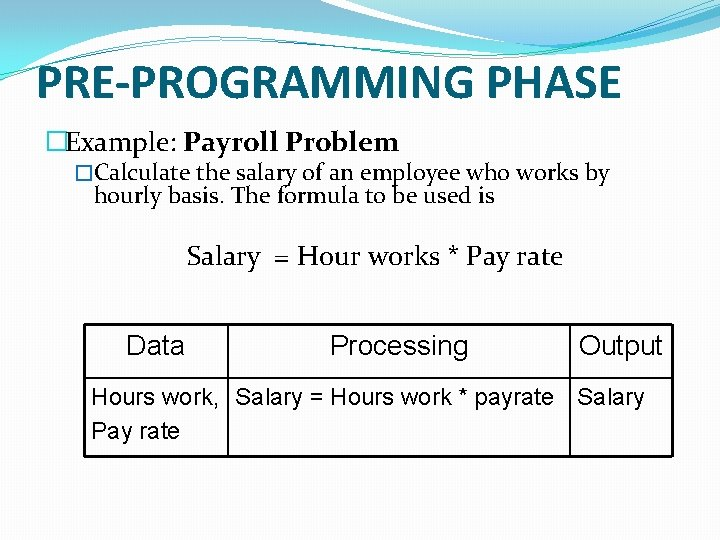PRE-PROGRAMMING PHASE �Example: Payroll Problem �Calculate the salary of an employee who works by hourly basis. The formula to be used is Salary = Hour works * Pay rate Data Processing Output Hours work, Salary = Hours work * payrate Salary Pay rateProblem 1 Write a Problem Analysis Chart (PAC) to convert the distance in miles to kilometers where 1. 609 kilometers per mile. Data Distance in miles Processing Output Kilometers = 1. 609 x miles Distance in kilometersProblem 2 �Write a Problem Analysis Chart (PAC) to find an area of a circle where area = pi * radius Data radius Processing area = 3. 14 x radius Output area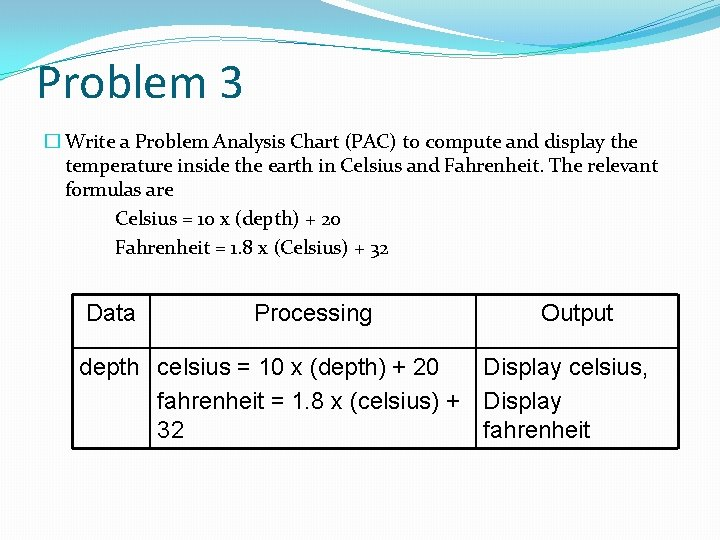Problem 3 � Write a Problem Analysis Chart (PAC) to compute and display the temperature inside the earth in Celsius and Fahrenheit. The relevant formulas are Celsius = 10 x (depth) + 20 Fahrenheit = 1. 8 x (Celsius) + 32 Data Processing Output depth celsius = 10 x (depth) + 20 Display celsius, fahrenheit = 1. 8 x (celsius) + Display 32 fahrenheit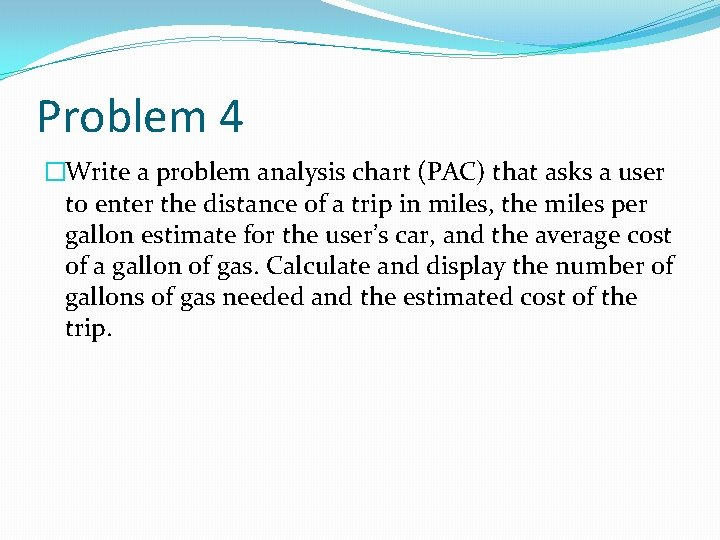Problem 4 �Write a problem analysis chart (PAC) that asks a user to enter the distance of a trip in miles, the miles per gallon estimate for the user’s car, and the average cost of a gallon of gas. Calculate and display the number of gallons of gas needed and the estimated cost of the trip.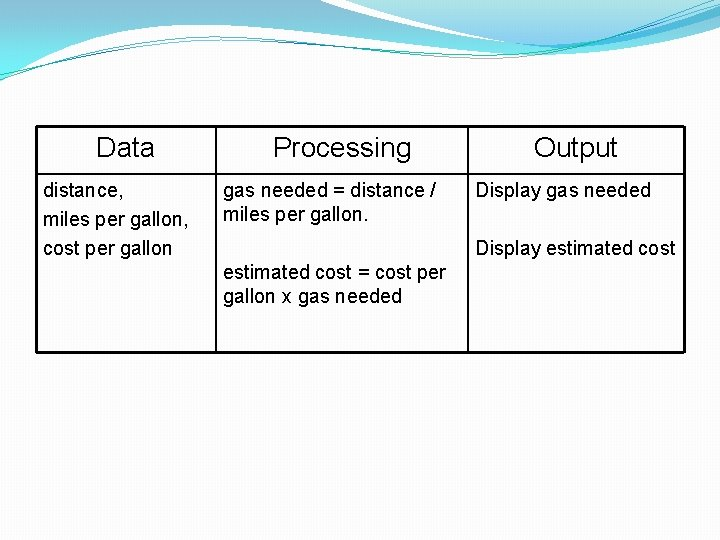Data distance, miles per gallon, cost per gallon Processing gas needed = distance / miles per gallon. Output Display gas needed Display estimated cost = cost per gallon x gas needed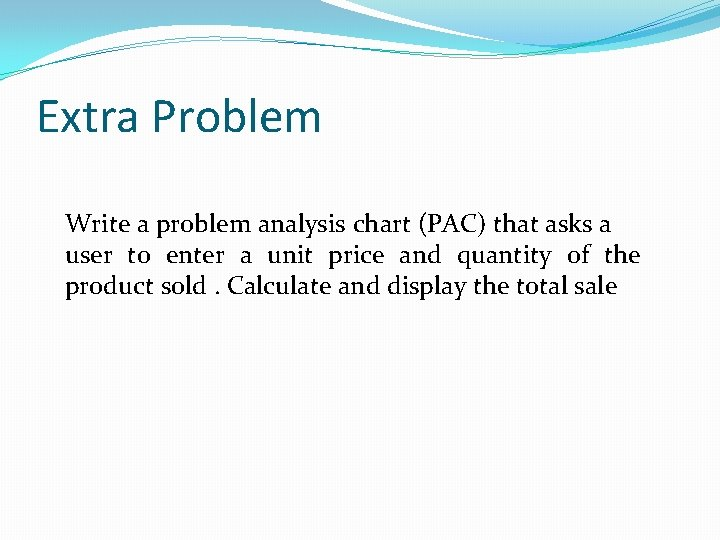Extra Problem Write a problem analysis chart (PAC) that asks a user to enter a unit price and quantity of the product sold. Calculate and display the total sale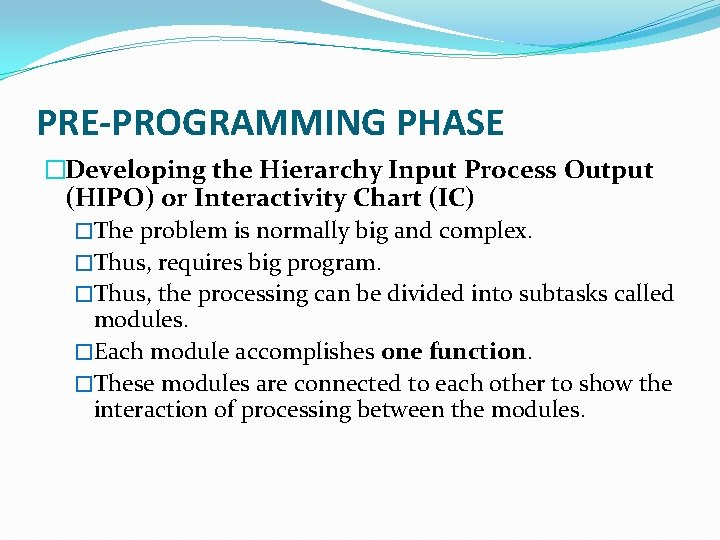PRE-PROGRAMMING PHASE �Developing the Hierarchy Input Process Output (HIPO) or Interactivity Chart (IC) �The problem is normally big and complex. �Thus, requires big program. �Thus, the processing can be divided into subtasks called modules. �Each module accomplishes one function. �These modules are connected to each other to show the interaction of processing between the modules.PRE-PROGRAMMING PHASE �Main/control module controls the flow all other modules. �The IC is developed using top-down-method: top to down left to right order (also refer to order of processing). �Modules are numbered, marked for duplication, repetition or decision.PRE-PROGRAMMING PHASE � The interaction will form a hierarchy, called Hierarchy Input Process Output Chart (HIPO) or Interactivity Chart (IC). Programming which use this approach (problem is divided into subtasks) is called Structured Programming. Main Module 1 Module 4 Module 2 Module 3 Module 5 Module 6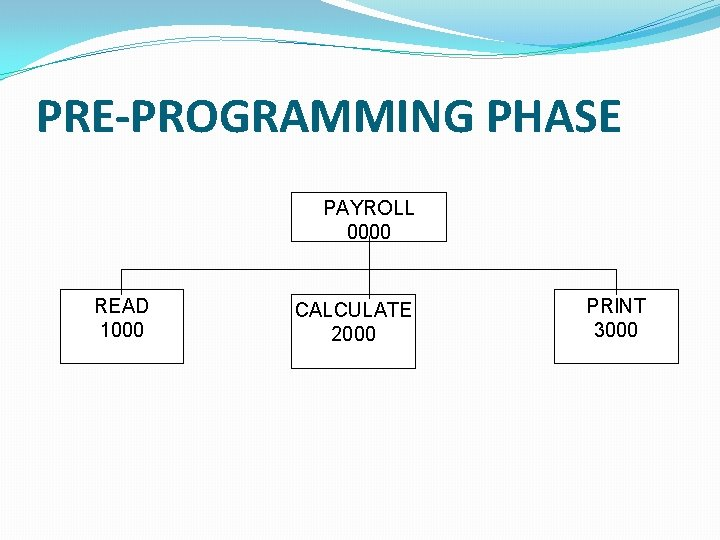PRE-PROGRAMMING PHASE PAYROLL 0000 READ 1000 CALCULATE 2000 PRINT 3000PRE-PROGRAMMING PHASE Example 2. 2: Extended Payroll Problem You are required to write a program to calculate both the gross pay and the net pay of every employee of your company. To determine the gross pay, you have to multiply the accumulated total hours worked by the employee, by the appropriate pay rate. The program should print the cheque that tells the total net pay. The net pay is calculated by subtracting the gross pay with any deductions that may be incurred by the employee.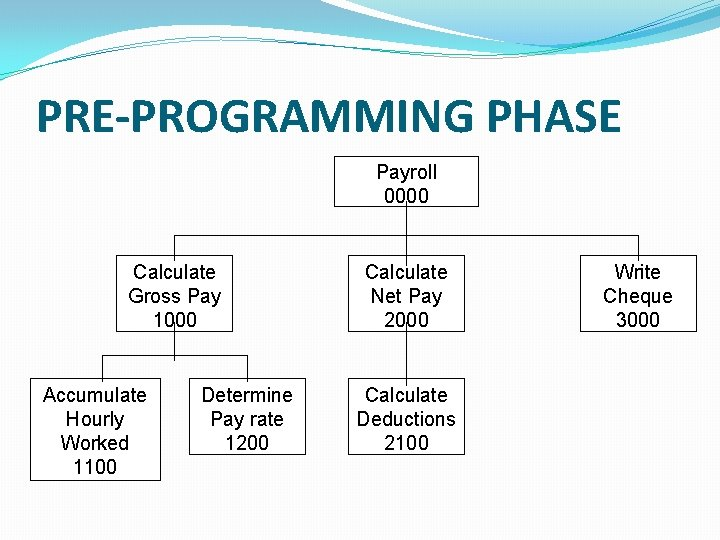PRE-PROGRAMMING PHASE Payroll 0000 Calculate Gross Pay 1000 Accumulate Hourly Worked 1100 Determine Pay rate 1200 Calculate Net Pay 2000 Calculate Deductions 2100 Write Cheque 3000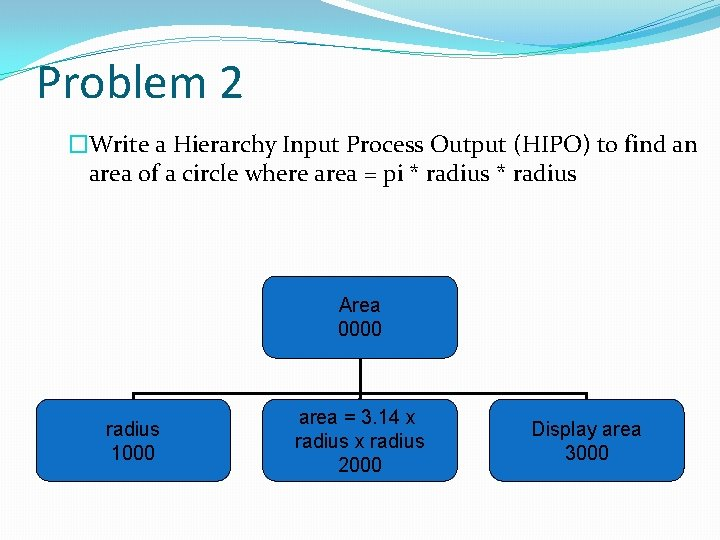Problem 2 �Write a Hierarchy Input Process Output (HIPO) to find an area of a circle where area = pi * radius Area 0000 radius 1000 area = 3. 14 x radius 2000 Display area 3000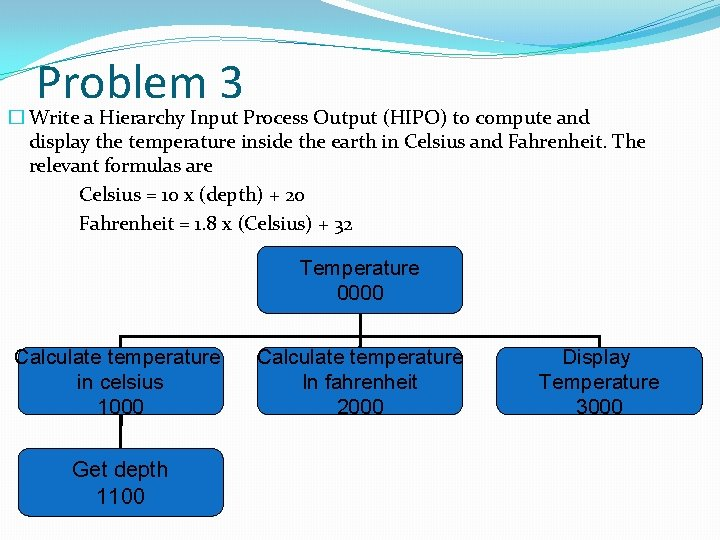Problem 3 � Write a Hierarchy Input Process Output (HIPO) to compute and display the temperature inside the earth in Celsius and Fahrenheit. The relevant formulas are Celsius = 10 x (depth) + 20 Fahrenheit = 1. 8 x (Celsius) + 32 Temperature 0000 Calculate temperature in celsius 1000 Get depth 1100 Calculate temperature In fahrenheit 2000 Display Temperature 3000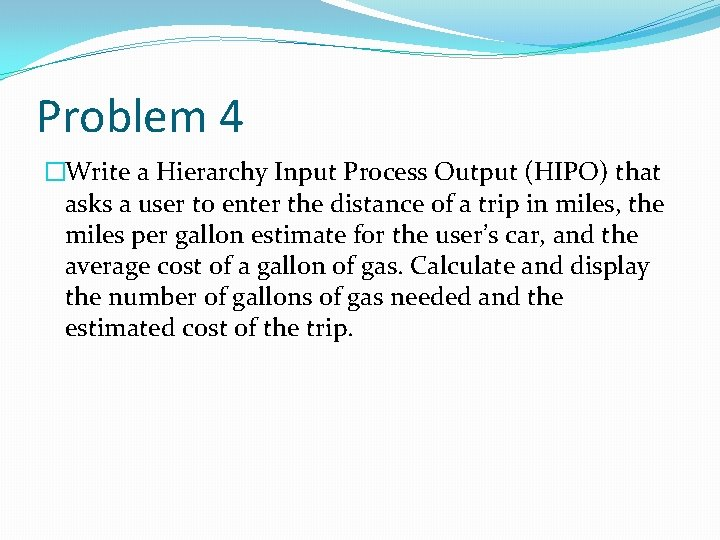Problem 4 �Write a Hierarchy Input Process Output (HIPO) that asks a user to enter the distance of a trip in miles, the miles per gallon estimate for the user’s car, and the average cost of a gallon of gas. Calculate and display the number of gallons of gas needed and the estimated cost of the trip.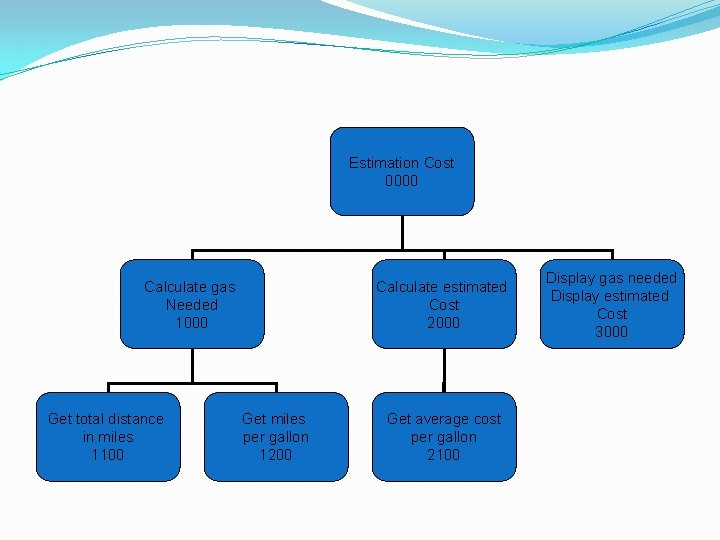Estimation Cost 0000 Calculate gas Needed 1000 Get total distance in miles 1100 Calculate estimated Cost 2000 Get miles per gallon 1200 Get average cost per gallon 2100 Display gas needed Display estimated Cost 3000Extra Problem Extended Extra Problem Find the total sale if the customer is given 20% discount on the sale itemsPRE-PROGRAMMING PHASE �Developing the Input Process Output (IPO) Chart �Extends and organizes the information in the Problem Analysis Chart. �It shows in more detail what data items are input, what are the processing or modules on that data, and what will be the result or output. �It combines information from PAC and HIPO Chart.PRE-PROGRAMMING PHASE IPO Chart Input All input data from PAC Processing Module All processing steps Module from HIPO / IC reference from the IC Output All output requirements from PACPRE-PROGRAMMING PHASE IPO for Extended Payroll Problem Input -Hours Worked -Pay Rate -Deduction Processing -Enter Hourly Worked -Enter Pay Rate -Calculate Gross Pay -Enter Deductions -Calculate Net Pay -Print Cheque -End Module Output 1100 1200 1000 2100 2000 3000 0000 -Net pay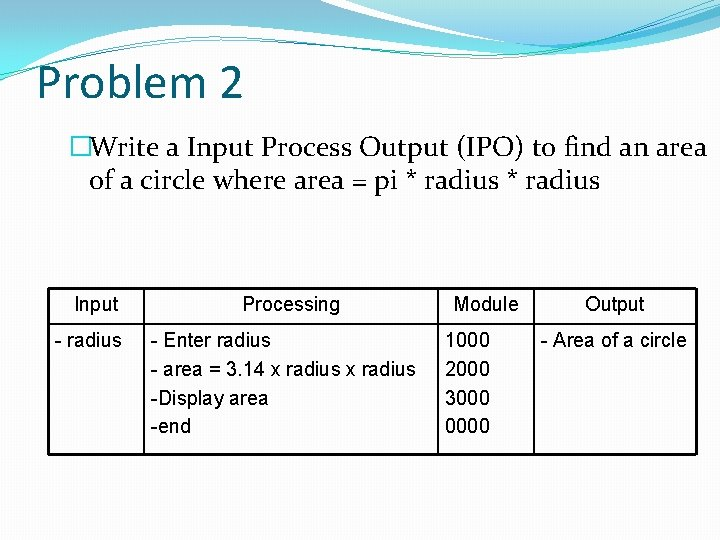Problem 2 �Write a Input Process Output (IPO) to find an area of a circle where area = pi * radius Input - radius Processing - Enter radius - area = 3. 14 x radius -Display area -end Module 1000 2000 3000 0000 Output - Area of a circleProblem 3 � Write an Input Process Output (IPO) to compute and display the temperature inside the earth in Celsius and Fahrenheit. The relevant formulas are Celsius = 10 x (depth) + 20 Fahrenheit = 1. 8 x (Celsius) + 32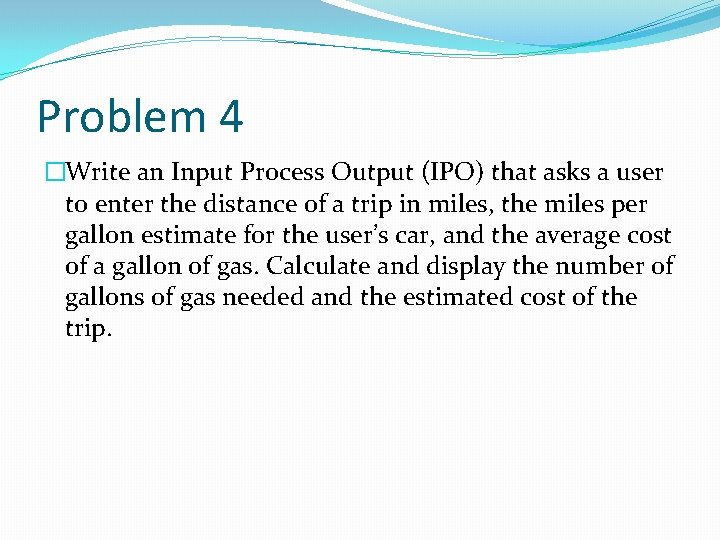Problem 4 �Write an Input Process Output (IPO) that asks a user to enter the distance of a trip in miles, the miles per gallon estimate for the user’s car, and the average cost of a gallon of gas. Calculate and display the number of gallons of gas needed and the estimated cost of the trip.Input - Distance in miles - Miles per gallon - Cost gas per gallon Processing - Enter distance - Enter miles per gallon - Calculate total gas needed - Enter cost gas per gallon - Calculate estimated cost - Display total gas and estimated cost - End Module 1100 1200 1000 2100 2000 3000 0000 Output -Total gas needed - Estimated cost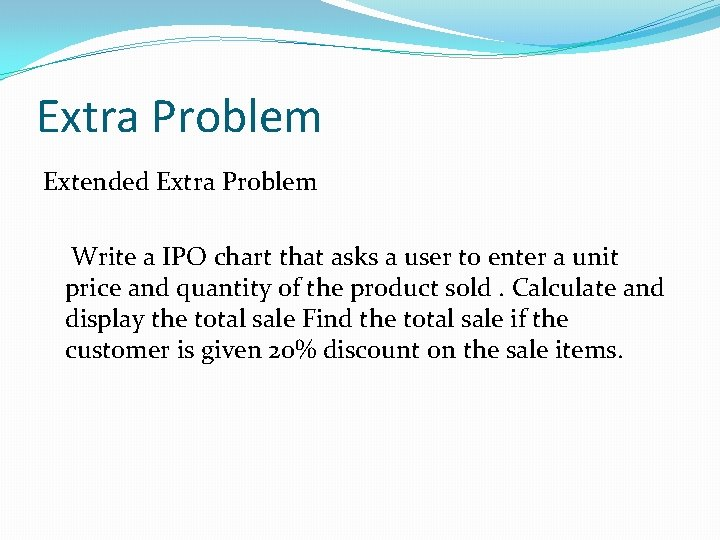Extra Problem Extended Extra Problem Write a IPO chart that asks a user to enter a unit price and quantity of the product sold. Calculate and display the total sale Find the total sale if the customer is given 20% discount on the sale items.